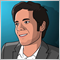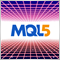# How to manage JPY pairs with parameters?700

Hi,

In previous version I use to do this way and it worked fine:

/// --- Prepare data --- ///
if (Symbol()=="AUDJPY" || Symbol()=="CADJPY" || Symbol()=="CHFJPY" || Symbol()=="EURJPY" || Symbol()=="GBPJPY" || Symbol()=="USDJPY" || Symbol()=="NZDJPY" )
{double Modify_StopLoss_v1 = Modify_StopLoss*100;  double Pips_Marge_v1 = Pips_Marge*100;  double Check_Spread_v1=Check_Spread*100; double Modify_Entry_v1 = Modify_Entry*100; double Daily_Candle_Pips_v1 = Daily_Candle_Pips*100;}

else  {Modify_StopLoss_v1 = Modify_StopLoss;  Pips_Marge_v1 = Pips_Marge;   Check_Spread_v1=Check_Spread;  Modify_Entry_v1 = Modify_Entry;  Daily_Candle_Pips_v1 = Daily_Candle_Pips;}

Now It says I have undeclared variables: so I did this way: I added "double"

/// --- Prepare data --- ///
if (Symbol()=="AUDJPY" || Symbol()=="CADJPY" || Symbol()=="CHFJPY" || Symbol()=="EURJPY" || Symbol()=="GBPJPY" || Symbol()=="USDJPY" || Symbol()=="NZDJPY" )
{double Modify_StopLoss_v1 = Modify_StopLoss*100;  double Pips_Marge_v1 = Pips_Marge*100;  double Check_Spread_v1=Check_Spread*100; double Modify_Entry_v1 = Modify_Entry*100; double Daily_Candle_Pips_v1 = Daily_Candle_Pips*100;}

else  {double Modify_StopLoss_v1 = Modify_StopLoss;  double Pips_Marge_v1 = Pips_Marge;   double Check_Spread_v1=Check_Spread;  double Modify_Entry_v1 = Modify_Entry;  double Daily_Candle_Pips_v1 = Daily_Candle_Pips;}

But it is not enough...as I call this variables in void_ontick()

Apparantly I must declare again this variables into parameters:

double Daily_Candle_Pips_v1 = 0.0045;
double Pips_Marge_v1 = 0.0005;

Here is my code:
//-----------------------------------------------------------------
// DAILY STRATEGY PRICE ACTION REVERSING... Pin bar?
//-----------------------------------------------------------------
double Daily_High = iHigh(Symbol(),PERIOD_D1,0);
double Daily_Low = iLow(Symbol(),PERIOD_D1,0);
double Daily_Open = iOpen(Symbol(),PERIOD_D1,0);

if (  Price_Action_Reversal && Hour()>=14 && Hour()<=22 && MathAbs(Bid-Daily_Open)<=Pips_Marge_v1 && Today_Profit_USD_News/(AccountBalance()-Today_Profit_USD_News)*100>=-Daily_Loss // If loss >= -0.5%
&&  ( (TimeDayOfWeek(TimeCurrent()) == 2) || (TimeDayOfWeek(TimeCurrent()) == 3) || (TimeDayOfWeek(TimeCurrent()) == 4)  || (TimeDayOfWeek(TimeCurrent()) == 5 && TimeDay(TimeCurrent())<=7)  ) ) {

// Long
Print("Price Action Reversal LONG: Actual Drawdown = %",Today_Profit_USD_News/(AccountBalance()-Today_Profit_USD_News)*100);
// Print("Print Day = ",TimeDay(OrderOpenTime()));
}

// Short
Print("Price Action Reversal Short: Actual Drawdown = %",Today_Profit_USD_News/(AccountBalance()-Today_Profit_USD_News)*100);}

}

double Daily_Open_Comment = iOpen(Symbol(),PERIOD_D1,0);
Comment("|--|<  Daily Opened Price = ",Daily_Candle_Pips_v1,"  >|--|<  Marge_Pips = ",Pips_Marge_v1,"  >|--|<  Today_Profit = €",Today_Profit_USD_News,"  >|--|<  DrawDown = %",Today_Profit_USD_News/(AccountBalance()-Today_Profit_USD_News)*100);

But if I backtest with GbbUsd it works...

But with JPY pairs... it doesn't convert my parameter with 2 zero less ?

RegardsModerator
13513

You could first declare the variables and then assign their values.

/// --- Prepare data --- ///
if (Symbol()=="AUDJPY" || Symbol()=="CADJPY" || Symbol()=="CHFJPY" || Symbol()=="EURJPY" || Symbol()=="GBPJPY" || Symbol()=="USDJPY" || Symbol()=="NZDJPY" )
{Modify_StopLoss_v1 = Modify_StopLoss*100;  Pips_Marge_v1 = Pips_Marge*100;  Check_Spread_v1=Check_Spread*100; Modify_Entry_v1 = Modify_Entry*100; Daily_Candle_Pips_v1 = Daily_Candle_Pips*100;}

else  {Modify_StopLoss_v1 = Modify_StopLoss;  Pips_Marge_v1 = Pips_Marge;   Check_Spread_v1=Check_Spread;  Modify_Entry_v1 = Modify_Entry;  Daily_Candle_Pips_v1 = Daily_Candle_Pips;}

Also note that you can find these JPY pairs and others like GOLD and etc.. by simply analyzing:

Digits();
 SYMBOL_DIGITS Digits after a decimal point int

So there is no need to hard code all the symbol names into the code and a simple multiplier related to digits can be a lot easier and will also work for symbols that you didn't include in your code.27896

1. Frenchytrader: Now It says I have undeclared variables: so I did this way: I added "double"
/// --- Prepare data --- ///
if (Symbol()=="AUDJPY" || Symbol()=="CADJPY" || Symbol()=="CHFJPY" || Symbol()=="EURJPY" || Symbol()=="GBPJPY" || Symbol()=="USDJPY" || Symbol()=="NZDJPY" )
{double Modify_StopLoss_v1 = Modify_StopLoss*100;  double Pips_Marge_v1 = Pips_Marge*100;  double Check_Spread_v1=Check_Spread*100; double Modify_Entry_v1 = Modify_Entry*100; double Daily_Candle_Pips_v1 = Daily_Candle_Pips*100;}

else  {double Modify_StopLoss_v1 = Modify_StopLoss;  double Pips_Marge_v1 = Pips_Marge;   double Check_Spread_v1=Check_Spread;  double Modify_Entry_v1 = Modify_Entry;  double Daily_Candle_Pips_v1 = Daily_Candle_Pips;}
But with JPY pairs... it doesn't convert my parameter with 2 zero less ?
Using strict mode, variables declared inside the braces "{}" are not visible after the closing brace. Thus your error and #1's suggestion.
2.  You are not adjusting SL, TP, and slippage for 4/5 digit brokers and for JPY pairs.
/// --- Prepare data --- ///
double   pip          = StringFind(_Symbol,"JPY") < 0 ? 0.01 : 0.0001;
int      pipDigits    = (int)MathLog10(pip/_Point);
int      pipsToPoints = int(pip / _Point);
int      slippage     = 3 * pipsToPoints;

double Modify_StopLoss_v1   = Modify_StopLoss   * pipsToPoints; // \   Or just drop all these
double Pips_Marge_v1        = Pips_Marge        * pipsToPoints; //  \  variables, and just use
double Modify_Entry_v1      = Modify_Entry      * pipsToPoints; //  /  instead of
double Daily_Candle_Pips_v1 = Daily_Candle_Pips * pipsToPoints; // /   Check_Spread_v1 * _Point

3. Marco vd Heijden: Also note that you can find these JPY pairs and others like GOLD and etc.. by simply analyzing:
The term pip is only defined for currencies, not metals. What is a TICK? - MQL4 forum  Analyzing digits or just looking for JPY will not work for exotics like USDMXN/USDZAR where the spread is beyond 300 pips. For that I've been trying
double dvpl = DeltaValuePerLot();
double pip  = 0.0001;
while(dvpl * pip < 5) pip *= 10;
Which gives the same results for most currencies, adjusts for the exotics, and should handle metals, i.e. a pip is worth about ¤10 (account currency.)700

Hello

Thank you guys : )